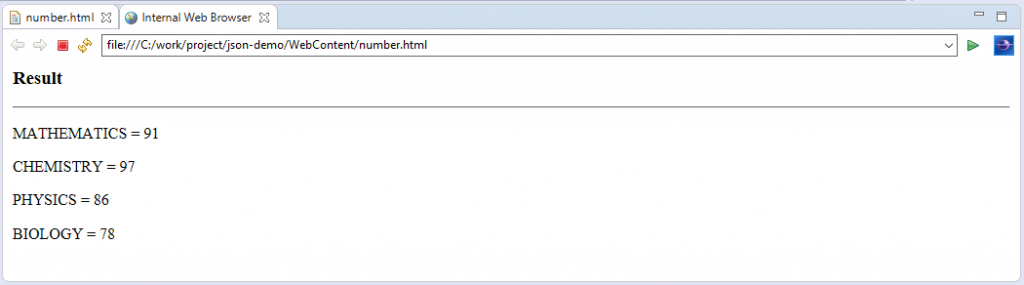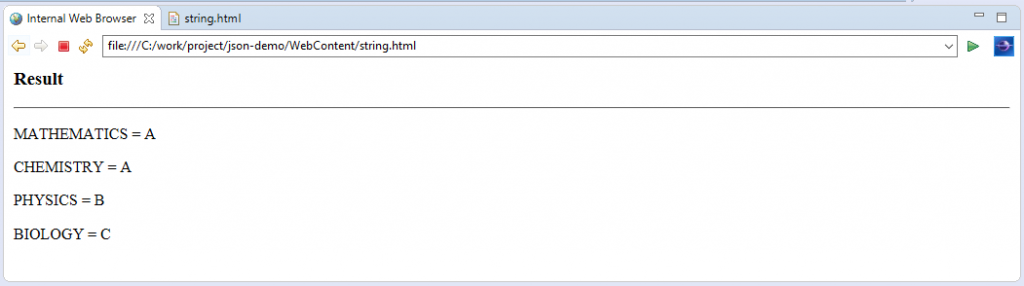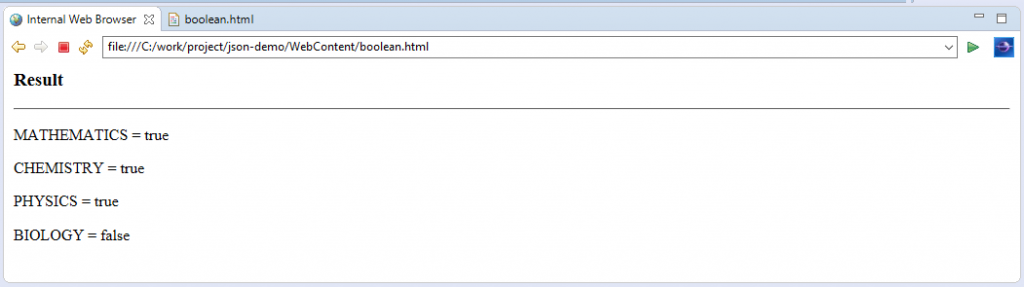# Learn about the Different JSON Data Types Format

0
7148In the last chapter, we discussed the basic syntaxes of JSON (JavaScript Object Notation) which are considered as a subset of the JavaScript syntax. In this chapter, we are going to discuss the various data types which are supported by JSON format.

JSON Data Types
The following are the data types which are supported by the JSON format.

 S No. Type Description 1. Number It is a JSON data type which is used to represent a number. It is a double- precision floating-point format in JavaScript. 2. String It is a JSON data type which is used to represent a string. It is a double-quoted Unicode with backslash escaping. 3. Boolean It is a JSON data type which is used to represent a boolean value. It can be either true or false. 4. Array It is a JSON data type which is used to represent an array (i.e. collection of multiple values in an ordered sequence). 5. Value In JSON format, it can be of a string type, a number type, a boolean type (i.e. true or false), a null type, etc. 6. Object It is a JSON data type which is represented as an unordered collection of key and value pairs. 7. Whitespace It is a JSON data type which can be used between any pair of tokens. 8. null It is a JSON data type which is used to represent an empty value.

JSON Data Type – NUMBER
In JSON, the number datatype has the following properties.

• Number has a double precision floating-point format in JavaScript.
• In JSON format, the Octal and hexadecimal formats are not defined or used.
• There is no use of NaN (Not a Number) or Infinity number in JSON.
• Numbers in JSON can be further classified into the following types.

 S No. Number Type Description 1. Integer Integers are numbers which are represented as digits 1-9 or 0. An integer can be either positive or negative. 2. Fraction Fractions are number such as .2, .7, etc. 3. Exponent Exponents are numbers such as e, e+, e-, E, E+, E-.

Syntax

`var number-json-obj-name = { string : a_number_value1, string : a_number_value2,.......}`

Example

```<html>
<title>JSON example</title>
<script language="javascript">
var subject_marks = {maths: 91, chemistry: 97, physics: 86, biology: 78};
document.write ("<h3>Result</h3>");
document.write ("<hr>");
document.write ("<p>MATHEMATICS = " + subject_marks.maths+"</p>");
document.write ("<p>CHEMISTRY = " + subject_marks.chemistry+"</p>");
document.write ("<p>PHYSICS = " + subject_marks.physics+"</p>");
document.write ("<p>BIOLOGY = " + subject_marks.biology+"</p>");
</script>
<body>
</body>
</html>```

Output
When we execute above HTML code on browser then we will observe the following output.JSON Data Type – STRING
In JSON, the string datatype has the following properties.

• String has a sequence of none or more than zero double quoted Unicode characters with backslash escaping.
• A character is a single character string which has a length as 1.
• JSON format permits to use the following special characters in string.

 S No. Number Type Description 1. “ Double quotation. 2. \ Backslash. 3. / Forward slash 4. B Backspace. 5. f Form feed. 6. n New line. 7. r Carriage return. 8. t Horizontal tab. 9. u Four hexadecimal digits.

Syntax

`var string-json-obj-name = { string : “a_string_value1”, string : “a_number_value2”,.......}`

Example

```<html>
<title>JSON example</title>
<script language="javascript">
var subject_grade = {maths: "A", chemistry: "A", physics: "B", biology: "C"};
document.write ("<h3>Result</h3>");
document.write ("<hr>");
document.write ("<p>MATHEMATICS = " + subject_grade.maths+"</p>");
document.write ("<p>CHEMISTRY = " + subject_grade.chemistry+"</p>");
document.write ("<p>PHYSICS = " + subject_grade.physics+"</p>");
document.write ("<p>BIOLOGY = " + subject_grade.biology+"</p>");
</script>
<body>
</body>
</html>```

Output
When we execute above HTML code on browser, then we will observe the following output.JSON Data Types – BOOLEAN
In JSON, the Boolean data type can have two values i.e. true or false.

Syntax

`var boolean-json-obj-name = { string : true, string : false,.......}`

Example

```<html>
<title>JSON example</title>
<script language="javascript">
var subject_pass = {maths: true, chemistry: true, physics: true, biology: false};
document.write ("<h3>Result</h3>");
document.write ("<hr>");
document.write ("<p>MATHEMATICS = " + subject_pass.maths+"</p>");
document.write ("<p>CHEMISTRY = " + subject_pass.chemistry+"</p>");
document.write ("<p>PHYSICS = " + subject_pass.physics+"</p>");
document.write ("<p>BIOLOGY = " + subject_pass.biology+"</p>");
</script>
<body>
</body>
</html>```

Output
When we execute above HTML code on browser, then we will observe the following output.JSON Data Types – ARRAY
In JSON, an array datatype has the following properties.

• An array is an ordered collection of values.
• An array is represented as an enclosure in square brackets i.e. begins with. [. and ends with.]…
• The values in an array are separated by, (comma).
• Indexing in an array can be started at 0 or 1.
• Arrays are recommended to be used when the key names are sequential integers.

Syntax

`[value1, value2, value3, …]`

Example

```{
"student": [
{
"rollnum": "01",
"subject": "Java Programming",
"name": "Mohit Singh",
"course": "BTech",
"university": "International University"
},
]
}```

JSON Data Types – OBJECT
In JSON, an object datatype has the following properties.

• It is JSON data type which is represented as an unordered collection of key and value pairs.
• Objects are enclosed with in the curly braces i.e. it starts with ‘{‘ and ends with ‘}’.
• Between each of the key and the value pair, a ‘:’ (colon) is present.
• The key and value pairs are separated by, (comma).
• Always, all keys must be strings and should be unique.
• Objects are recommended to be used when the key names are arbitrary strings.

Syntax

`{String: value1, string: value2,…}`

Example

```	{
"rollnum": "01",
"subject": "Java Programming",
"name": "Mohit Singh",
"course": "BTech",
"university": "International University"
}```

JSON Data Types – WHITESPACE
It is a JSON data type which can be used between any pair of tokens. They are used to make the code more readable.

Syntax

`{string:" ",…}`

Example
In the following example, we are going to demonstrate two declarations i.e. with and without whitespace.

```{
"subject": "Java Programming",
"name": "MohitSingh",
}```

JSON Data Types – NULL
It is a JSON data type which is used to represent an empty value.

Syntax

`null`

Example

```{
"subject": null,
}```

JSON Data Types – VALUE
In JSON, a value could be a Number, String, Array, object, Boolean or Null.

Syntax

`String | Number | Object | Array | true | false | null`

Example

```var subject_grade = {maths: "A", chemistry: "A", physics: "B", biology: "C"};
var subject_marks = {maths: 91, chemistry: 97, physics: 86, biology: 78};
var subject_pass = {maths: true, chemistry: true, physics: true, biology: false};```

Source code for the various data types of json format

Conclusion: –
In this chapter, we discussed about the various data types available in JSON format along with the suitable examples.